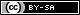# [EN] [CTF] TAMU - Simple DES

Write-up for challenge 'Simple DES' (Crypto, 125 pts) from TAMU CTF 2018.

3 minutes de lecture

Larry is working on an encryption algorithm based on DES.

He hasn’t worked out all the kinks yet, but he thinks it works.

Your job is to confirm that you can decrypt a message, given the algorithm and parameters used.

His system works as follows:

1. Choose a plaintext that is divisible into 12bit ‘blocks’
2. Choose a key at least 8bits in length
3. For each block from `i=0` while `i<N` perform the following operations
4. Repeat the following operations on block `i`, from `r=0` while `r<R`
5. Divide the block into 2 6bit sections `Lr`,`Rr`
6. Using `Rr`, “expand” the value from 6bits to 8bits.
Do this by remapping the values using their index, e.g.
1 2 3 4 5 6 -> 1 2 4 3 4 3 5 6
7. XOR the result of this with 8bits of the `Key` beginning with `Key[iR+r]` and wrapping back to the beginning if necessary.
8. Divide the result into 2 4bit sections `S1`, `S2`
9. Calculate the 2 3bit values using the two “S boxes” below, using S1 and S2 as input respectively.
S1 0 1 2 3 4 5 6 7
0 101 010 001 110 011 100 111 000
1 001 100 110 010 000 111 101 011
S2 0 1 2 3 4 5 6 7
0 100 000 110 101 111 001 011 010
1 101 011 000 111 110 010 001 100
1. Concatenate the results of the S-boxes into 1 6bit value
2. XOR the result with `Lr`
3. Use `Rr` as `Lr` and your altered `Rr` (result of previous step) as `Rr` for any further computation on block `i`
4. increment `r`

He has encryped a message using Key="Mu”, and R=2. See if you can decipher it into plaintext.

Submit your result to Larry in the format Gigem{plaintext}.

Binary of ciphertext: `01100101 00100010 10001100 01011000 00010001 10000101`

Since the key and the number of iteration are known, we simply need to build a decryption function to which we will give the ciphertext. Based on Larry’s encryption algorithm, here are the different steps needed to decrypt a message :

1. Divide the encrypted text into 12-bit blocks
2. For each block from `i=0` while `i<N` perform the following operations
3. Repeat the following operations on block `i`, from `r=R-1` while `r<=0`
4. Divide the block into 2 6bit sections `Rr` (left) and `Rr-alt` (right)
5. Using `Rr`, “expand” the value from 6bits to 8bits using the same method as encryption (e.g. 1 2 3 4 5 6 -> 1 2 4 3 4 3 5 6)
6. XOR the result of this with 8bits of the `Key` beginning with `Key[iR+r]` and wrapping back to the beginning if necessary.
7. Divide the result into 2 4bit sections `S1`, `S2` and calculate the 2 3bit values using the two “S boxes” above, using S1 and S2 as input respectively.
8. Concatenate the results of the S-boxes into 1 6bit value
9. XOR the result with `Rr-alt` to recover `Lr`
10. Your new block consists of `Lr`, the result of the XOR above (left) and` Rr` (right)
11. Decrement `r`
 ``````1 2 3 `````` ``````[+] Cipher: 01100101 00100010 10001100 01011000 00010001 10000101 [+] Flag: 01001101 01101001 01001110 00110000 01101110 00100001 Gigem{MiN0n!}``````Cet article est mis à disposition selon les termes de la Licence Creative Commons Attribution-ShareAlike 4.0 International. Cet article a été publié il y a 802 jours, son contenu peut être inexact, voire erroné, et l'application des conseils ou consignes présents dans cet article doit être fait à votre propre appréciation. L'auteur de l'article ne pourra être tenu responsable des inconvénients pouvant résulter de l'application des conseils et consignes énoncés dans cet article.